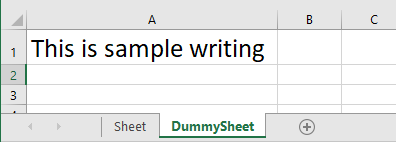## Excel Spread Sheets with python

First, let’s go over some basic definitions:
An Excel spreadsheet document is called a workbook. A single workbook is saved in a file with the .xlsx extension.

Each workbook can contain multiple sheets (also called worksheets). The sheet the user is currently viewing (or last viewed before closing Excel) is called the active sheet.

Each sheet has columns (addressed by letters starting at A) and rows (addressed by numbers starting at 1). A box at a particular column and row is called a cell.

Each cell can contain a number or text value. The grid of cells with data makes up a sheet.

## OpenPyXL Module with python selenium

OpenPyXL is a library used to read and write Excel 2010 xlsx/xlsm/xltx/xltm files. This is the library we will be using in this tutorial to work with Excel documents.

The first thing we need to do in order to make use of this library is to install OpenPyXL. Python does not come with OpenPyXL, so you’ll have to install it.

``pip install openpyxl``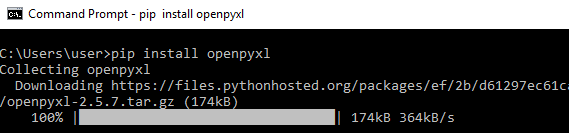## Read Excel using OpenpyXl with Python selenium

Post installing the OpenPyXl, let's start to read an excel file from the system. For example, purpose, I will be using the excel sheet data in the below image.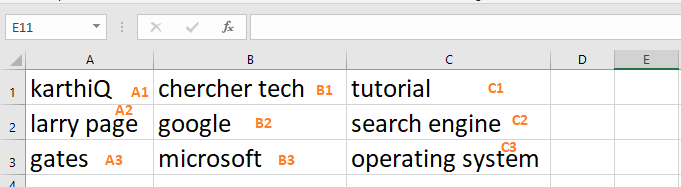#### Steps to read excel using OpenPyXl :

• Import the load_workbook function from openpyxl module, load_workbook helps to load the excel file into memory
• Get the active worksheet from the excel file, and an excel file is like a tree, and the worksheet is like a leaf. So an excel file can contain n-number of sheets
• get the cell by using the column name with a Row number, for example, sheet start with A1, A1 means that Column name is A and the row number is 1
• You also get the cell using row, and column number, row, and column number starting with 1. A1 is equivalent to row=1, column=1
``````import unittest

class Test(unittest.TestCase):

# set file path
filepath="excel-example.xlsx"
# activate demo.xlsx
sheet=wb.active
# get b1 cell value
b1=sheet['B1']
print("b1 --> ", b1.value)
# get b2 cell value
b2=sheet['B2']
print("b2 --> ", b2.value)
# get b3 cell value
b3=sheet.cell(row=3,column=2)
print("b3 --> ", b3.value)

if __name__ == "__main__":
unittest.main()``````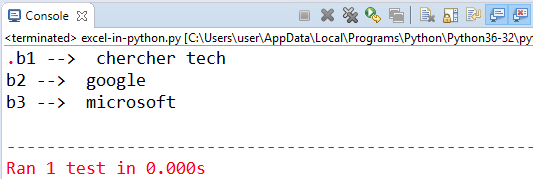## Get All values of a specific column

We can retrieve fetch the values present in the specific column using openpyxl in python.

• Import the load_workbook from openpyxl
• Get the sheet using the name of the sheet, careful about CASE
• Get the column which you want to iterate
• Using for loop go till the last value
• Print the values inside the loop
``````import unittest

class Test(unittest.TestCase):

# set file path
filepath="excel-example.xlsx"
sheet = wb["Sheet1"]

row_count= sheet.max_row
print("Total rows : ", row_count)
for cell in sheet['C']:
print(cell.value)

if __name__ == "__main__":
unittest.main()``````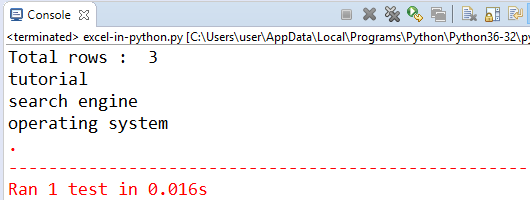## Write Excel file using openpyxl in python selenium

We can create an excel file using an openpyxl module in python.

#### Steps to writing excel file :

• Import the openpyxl and load_workbook
• Create an object to Workbook class and store it in a variable, this helps to create an Excel file
• create_sheet() function creates a new sheet in the above created excel file, you need to pass sheet name, and at what index you want to create the sheet, the index starts with 0
• Using sheet["A1"].value, we can set the value to the cell of the excel sheet.
• You must save the excel file to write the values to the excel and the local file system.
``````import unittest
import openpyxl

class Test(unittest.TestCase):

wb = openpyxl.Workbook()
sheet = wb.create_sheet("DummySheet", 1)
sheet["A1"].value = "This is sample writing"
wb.save("test-excel.xlsx")

if __name__ == "__main__":
unittest.main()``````